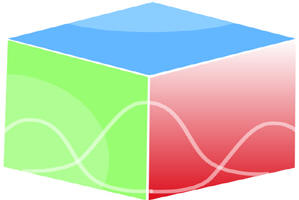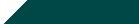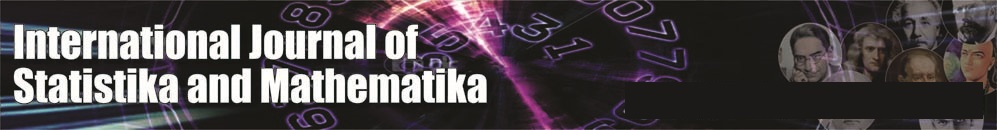``Home| Journals | Statistics Online Expert | About Us | Contact UsUntitled Document

International Journal of Statistika and Mathematika, ISSN: 2277- 2790 E-ISSN: 2249-8605

Volume 3, Issue 1, 2012 pp 27-33

Research Article

Some Common Fixed Point Theorem for Cone Metric Space

Shailesh T.Patel1, Ramakant Bhardwaj2

Research Scholar of Singhania University, Pacheri Bari, Jhunjhunu (RJ) INDIA

Truba Institutions of Engineering & I.T. Bhopal, (MP) INDIA

Abstract

In this paper, we proof some fixed point and common fixed point theorem for Cone metric space.
Let X be a Real banach space and P a subset of X.P is called a cone if P satisfy followings conditions:

1. P is closed, non empty and P≠0
2. Ax + By Є P for all x,y Є P and non negative real numbers a,b
3. P ∩ (-P) = { 0 }

Given a cone P  X, we define a partial ordering ≤ on X with respect to P by y-x Є P.
We shall write x << y if (y-x) Є int P, denoted by  the norm on X. the cone P is called normal if there is a number k > 0 such that for all x,y Є X
0 ≤ x ≤ y                                      ………………..(A)
The least positive number k satisfying the above condition (A) is called the normal constant of P.
The authors showed that there is no normal cones with normal constant M < 1 and for each k > 1
There are cone with normal constant M > k.
The cone P is called regular if every increasing sequence which is bounded from the above is convergent, that is if {xn}n≥1  is a sequence such that x1 ≤ x2 ≤ …..≤ y for some y Є X,
Then there is x Є X .
The cone P is regular iff every decreasing sequence which is bounded from below is convergent.